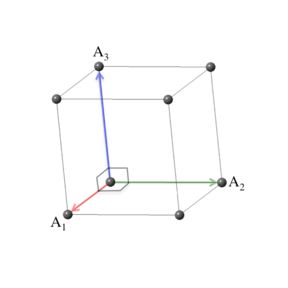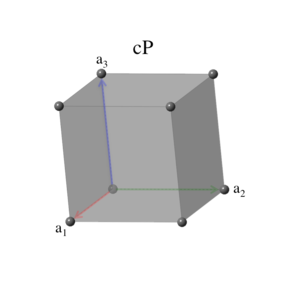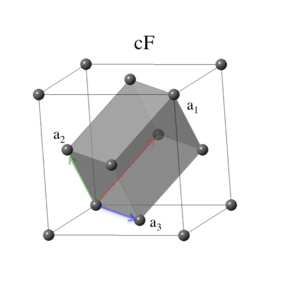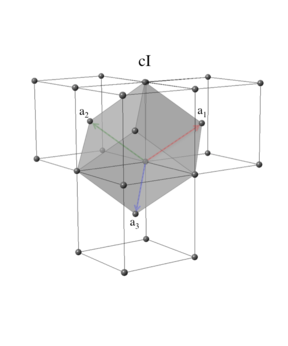# Encyclopedia of Crystallographic Prototypes

M. J. Mehl, D. Hicks, C. Toher, O. Levy, R. M. Hanson, G. L. W. Hart, and S. Curtarolo, The AFLOW Library of Crystallographic Prototypes: Part 1, Comp. Mat. Sci. 136, S1-S828 (2017). (doi=10.1016/j.commatsci.2017.01.017)
D. Hicks, M. J. Mehl, E. Gossett, C. Toher, O. Levy, R. M. Hanson, G. L. W. Hart, and S. Curtarolo, The AFLOW Library of Crystallographic Prototypes: Part 2, Comp. Mat. Sci. 161, S1-S1011 (2019). (doi=10.1016/j.commatsci.2018.10.043)

## The Cubic Crystal SystemThe cubic crystal system is defined as having the symmetry of a cube: the conventional unit cell can be rotated by 90$^\circ$ about any axis, or by 180$^\circ$ around an axis running through the center of two opposing cube edges, or by 120$^\circ$ around a body diagonal, and retain the same shape. The conventional cell then takes the form \begin{eqnarray} \mathbf{A}_1 & = & a \, \mathbf{\hat{x}} \nonumber \\ \mathbf{A}_2 & = & a \, \mathbf{\hat{y}} \nonumber \\ \mathbf{A}_3 & = & a \, \mathbf{\hat{z}}, \end{eqnarray} with unit cell volume \begin{equation} V = a^3. \end{equation}
This is the limiting case of both the orthorhombic and tetragonal systems when all primitive vectors are equal in length. There are three Bravais lattices in the cubic system.

### Lattice 12: Simple CubicThe simple cubic system is identical to the conventional cubic unit cell \begin{eqnarray} \mathbf{a}_1 & = & a \, \mathbf{\hat{x}} \nonumber \\ \mathbf{a}_2 & = & a \, \mathbf{\hat{y}} \nonumber \\ \mathbf{a}_3 & = & a \, \mathbf{\hat{z}}, \end{eqnarray} with volume \begin{equation} V = a^3. \end{equation}
This can also be considered as a rhombohedral lattice with $\alpha = \pi/2$. The space groups associated with this lattice are \begin{array}{lll} 195. ~ \mbox{P23} & 198. ~ \mbox{P2$_{1}$3} & 200. ~ \mbox{Pm$\overline{3}$} \\ 201. ~ \mbox{Pn$\overline{3}$} & 205. ~ \mbox{Pa$\overline{3}$} & 207. ~ \mbox{P432} \\ 208. ~ \mbox{P4$_{2}$32} & 212. ~ \mbox{P4$_{3}$32} & 213. ~ \mbox{P4$_{1}$32} \\ 215. ~ \mbox{P$\overline{4}$3m} & 218. ~ \mbox{P$\overline{4}$3n} & 221. ~ \mbox{Pm$\overline{3}$m} \\ 222. ~ \mbox{Pn$\overline{3}$n} & 223. ~ \mbox{Pm$\overline{3}$n} & 224. ~ \mbox{Pn$\overline{3}$m} \\ \end{array}

### Lattice 13: Face-Centered CubicThe face-centered cubic lattice has the same periodicity as its simple cubic parent with the addition of a translation from one corner of the cube to the center of any face. Our standard face-centered cubic primitive vectors have the form \begin{eqnarray} \mathbf{a}_1 & = & \frac{a}{2} \, \mathbf{\hat{y}} + \frac{a}{2} \, \mathbf{\hat{z}} \\ \mathbf{a}_2 & = & \frac{a}{2} \, \mathbf{\hat{x}} + \frac{a}{2} \, \mathbf{\hat{z}} \\ \mathbf{a}_3 & = & \frac{a}{2} \, \mathbf{\hat{x}} + \frac{a}{2} \, \mathbf{\hat{y}}, \end{eqnarray} and the primitive cell volume is \begin{equation} V = \frac{a^3}{4}. \end{equation}
There are four face-centered cubic primitive cells in the conventional cubic cell. The face-centered cubic lattice can be considered as a rhombohedral lattice where $\alpha = 60^\circ$. The space groups associated with this lattice are \begin{array}{lll} 196. ~ \mbox{F23} & 202. ~ \mbox{Fm$\overline{3}$} & 203. ~ \mbox{Fd$\overline{3}$} \\ 209. ~ \mbox{F432} & 210. ~ \mbox{F4$_{1}$32} & 216. ~ \mbox{F$\overline{4}$3m} \\ 219. ~ \mbox{F4$\overline{3}$c} & 225. ~ \mbox{Fm$\overline{3}$m} & 226. ~ \mbox{Fm$\overline{3}$c} \\ 227. ~ \mbox{Fd$\overline{3}$m} & 228. ~ \mbox{Fd$\overline{3}$c} & \\ \end{array}

### Lattice 14: Body-Centered CubicLike its predecessors in the orthorhombic and tetragonal systems, the body-centered cubic crystal has the same periodicity as its parent with the addition of a translation from one corner of the cube to its center. Our standard body-centered cubic primitive vectors have the form \begin{eqnarray} \mathbf{a}_1 & = & - \frac{a}{2} \, \mathbf{\hat{x}} + \frac{a}{2} \, \mathbf{\hat{y}} + \frac{a}{2} \, \mathbf{\hat{z}} \\ \mathbf{a}_2 & = & ~ \frac{a}{2} \, \mathbf{\hat{x}} - \frac{a}{2} \, \mathbf{\hat{y}} + \frac{a}{2} \, \mathbf{\hat{z}} \\ \mathbf{a}_3 & = & ~ \frac{a}{2} \, \mathbf{\hat{x}} + \frac{a}{2} \, \mathbf{\hat{y}} - \frac{a}{2} \, \mathbf{\hat{z}}, \end{eqnarray} and the primitive cell volume is \begin{equation} V = \frac{a^3}{2}. \end{equation}
There are two body-centered cubic primitive cells in the conventional cubic cell. The body-centered cubic lattice can be considered as a rhombohedral lattice where $\alpha = \cos^{-1} (-1/3) \approx 109.47^\circ$. The space groups associated with this lattice are \begin{array}{lll} 197. ~ \mbox{I23} & 199. ~ \mbox{I2$_{1}$3} & 204. ~ \mbox{Im$\overline{3}$} \\ 206. ~ \mbox{Ia$\overline{3}$} & 211. ~ \mbox{I432} & 214. ~ \mbox{I4$_{1}$32} \\ 217. ~ \mbox{I$\overline{4}$3m} & 220. ~ \mbox{I$\overline{4}$3d} & 229. ~ \mbox{Im$\overline{3}$m} \\ 230. ~ \mbox{Ia$\overline{3}$d} & ~ & ~ \\ \end{array}